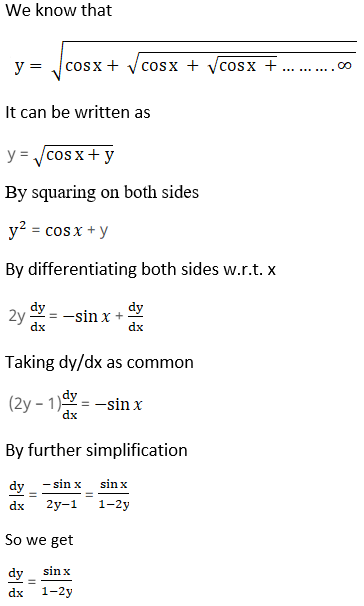# RS Aggarwal Solutions for Class 12 Maths Chapter 10: Differentiation Exercise 10G

Derivatives of an Infinite Series is the concept which is covered under the Exercise 10G of Chapter 10. Various solved examples are available before the exercise wise problems to help students analyse the problems that would appear in the board exam. The solutions designed by faculty at BYJU’S contain explanations to make it easier for the students while studying. Students who wish to score well in the board exams can download RS Aggarwal Solutions Class 12 Maths Chapter 10 Differentiation Exercise 10G free PDF, from the links provided below.

## RS Aggarwal Solutions for Class 12 Maths Chapter 10: Differentiation Exercise 10G Download PDF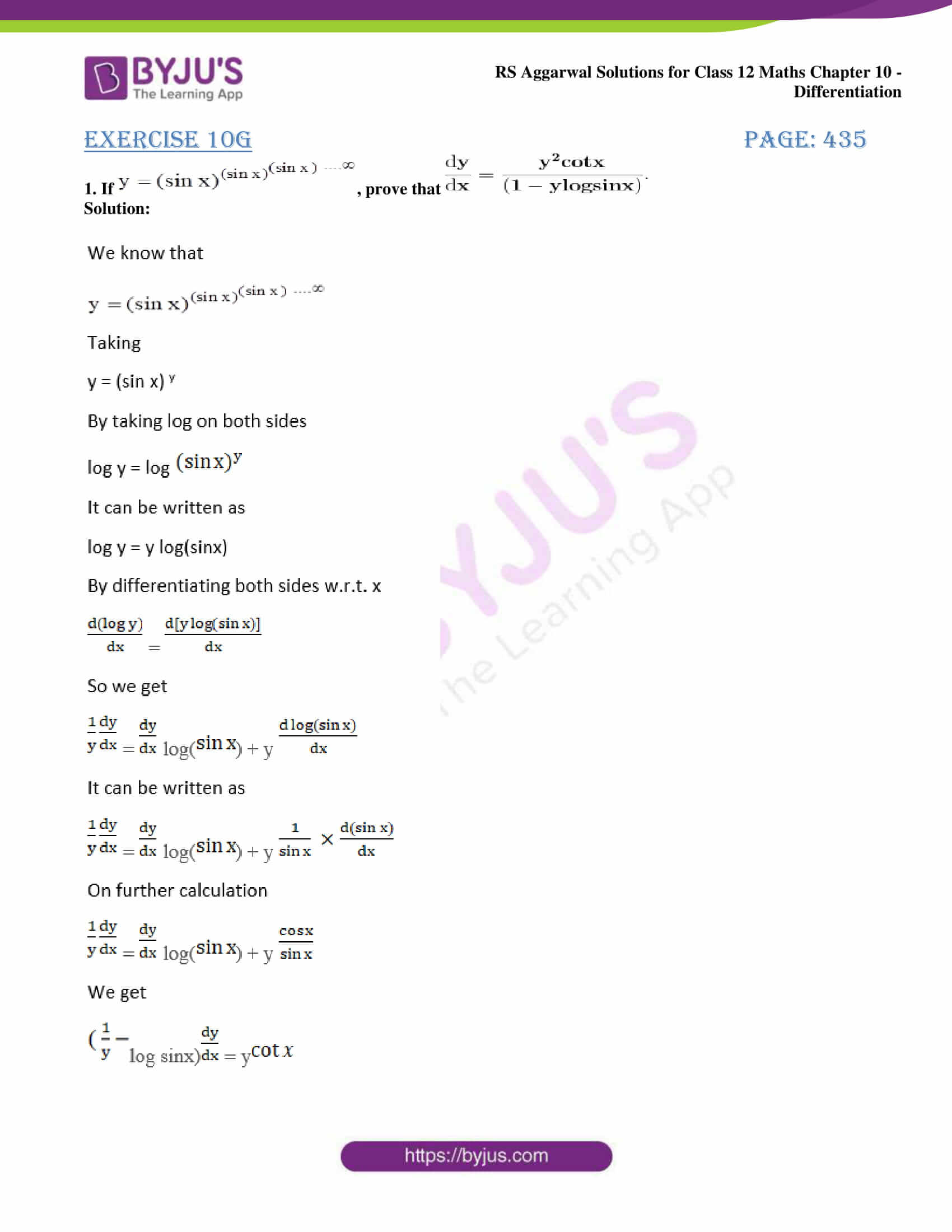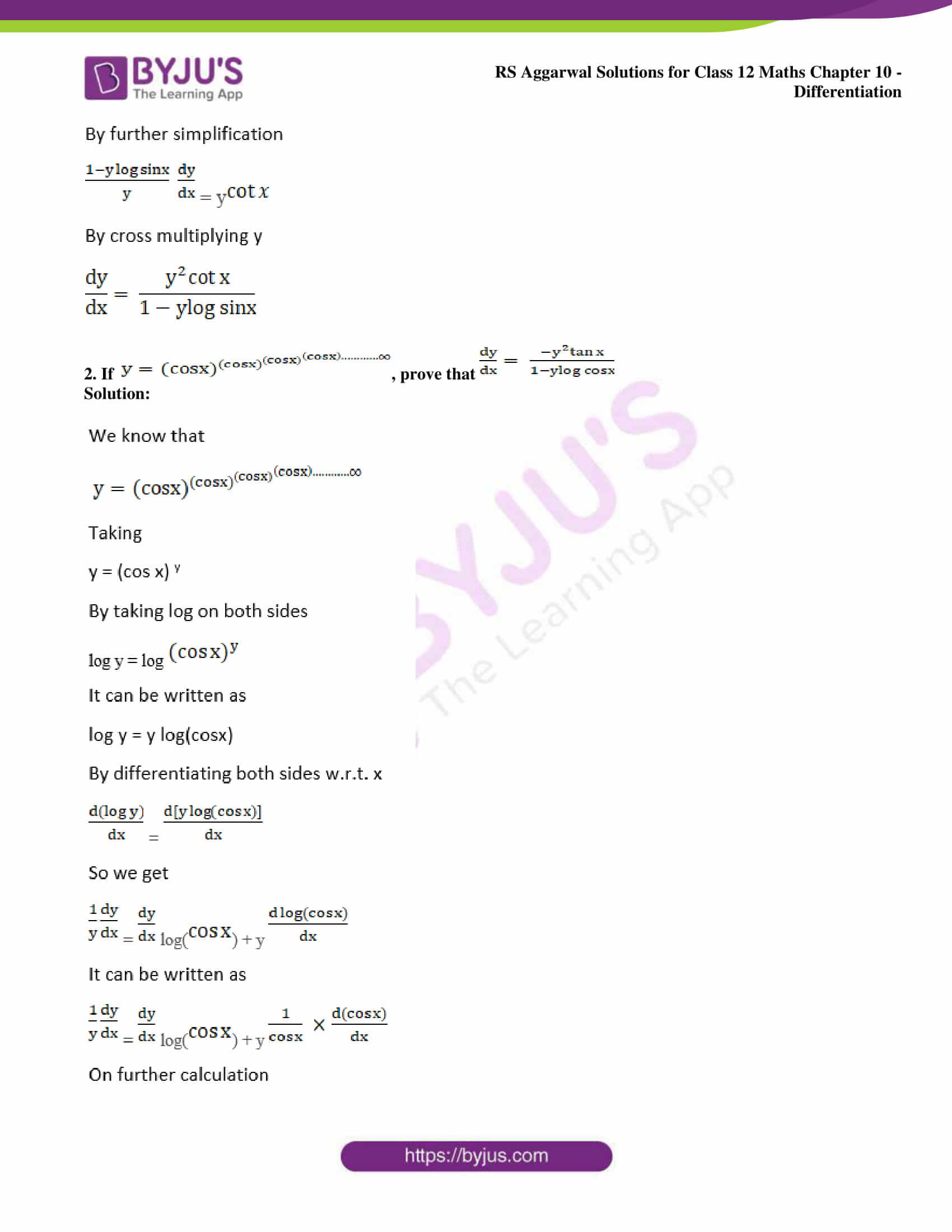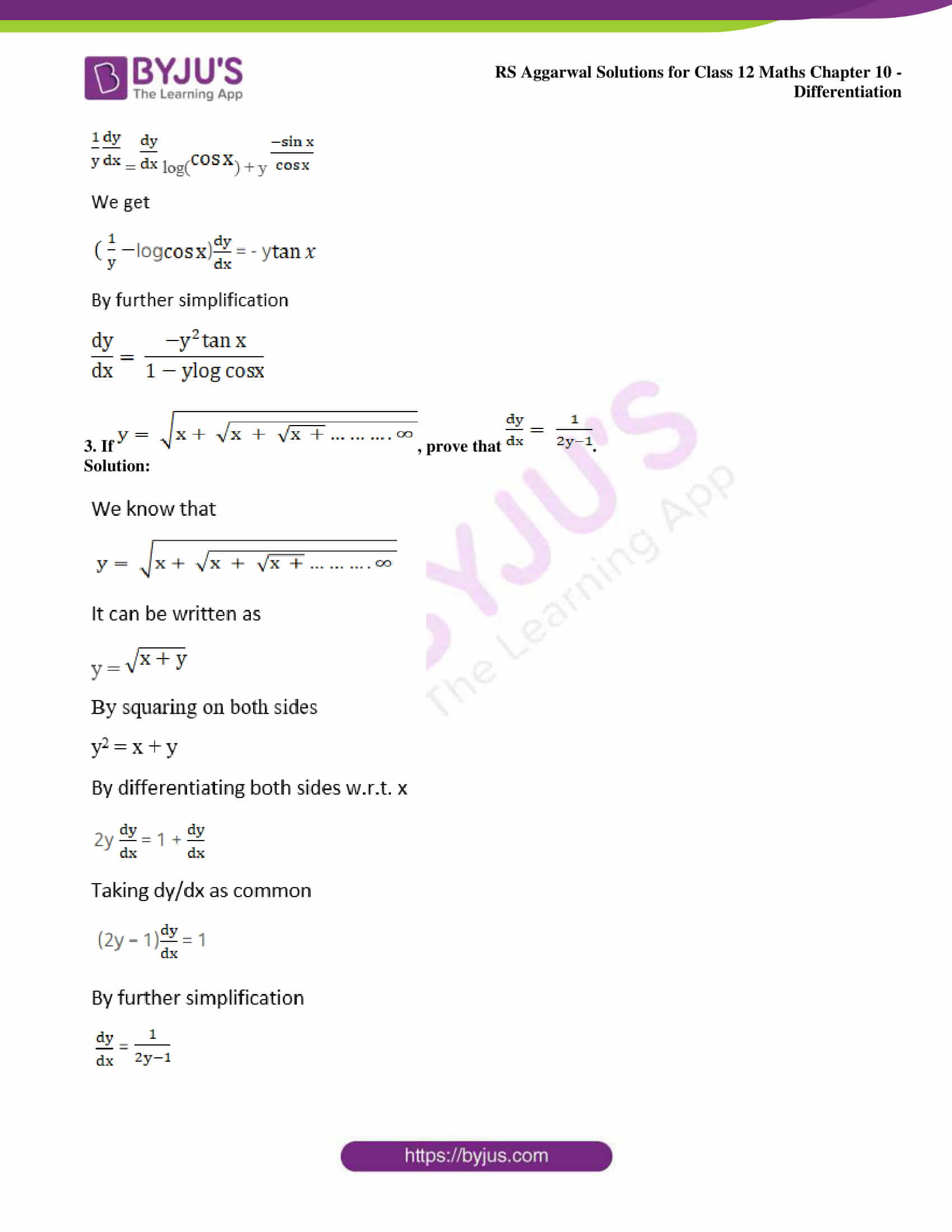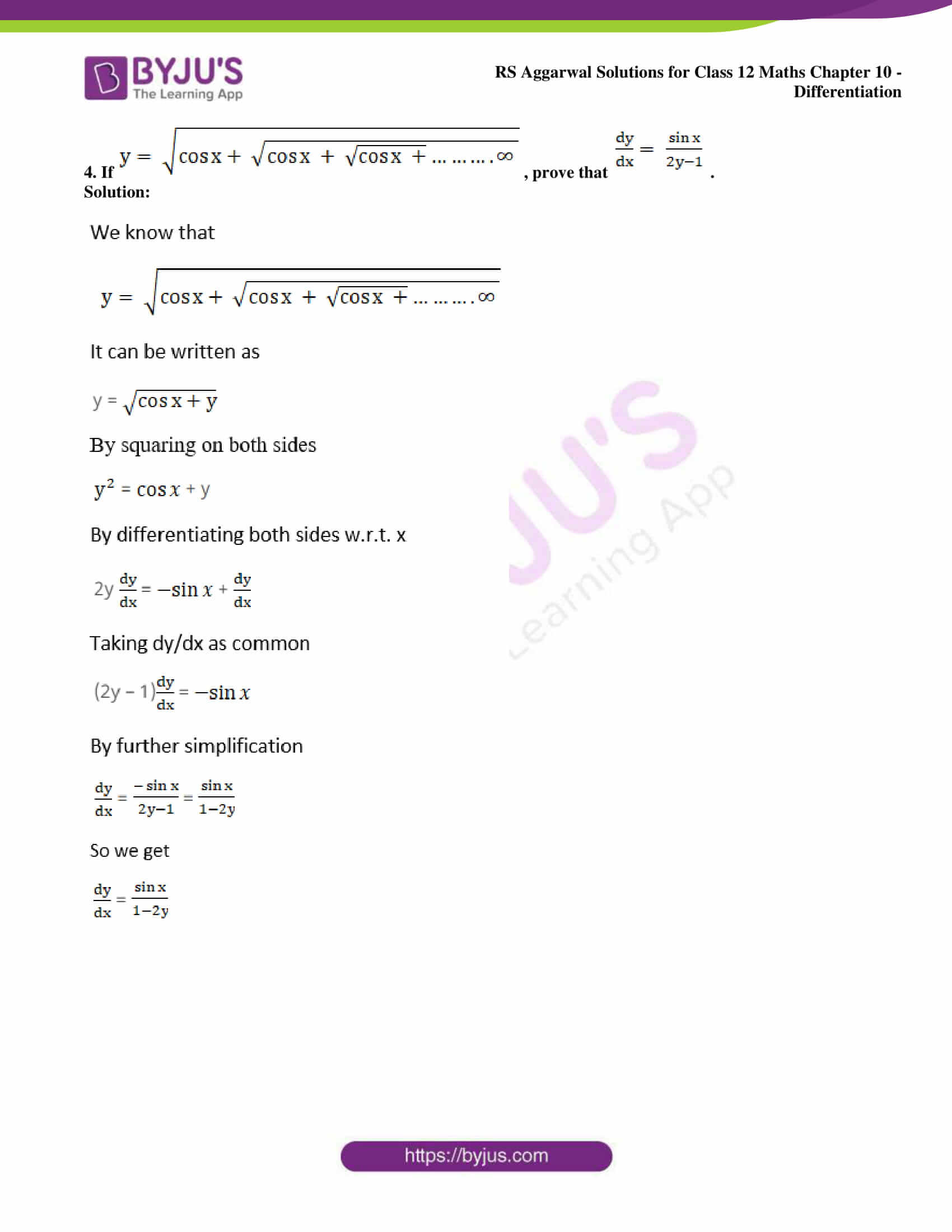### Access other exercise solutions of Class 12 Maths Chapter 10: Differentiation

Exercise 10A Solutions

Exercise 10B Solutions

Exercise 10C Solutions

Exercise 10D Solutions

Exercise 10E Solutions

Exercise 10F Solutions

Exercise 10H Solutions

Exercise 10I Solutions

Exercise 10J Solutions

### Access RS Aggarwal Solutions for Class 12 Maths Chapter 10: Differentiation Exercise 10G

1. If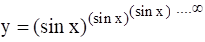, prove that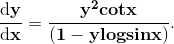Solution: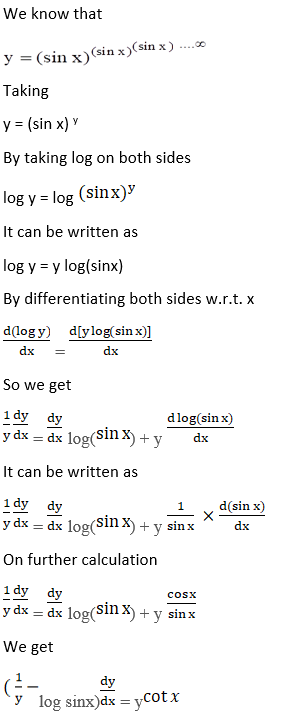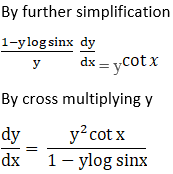2. If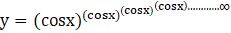, prove that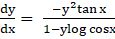Solution: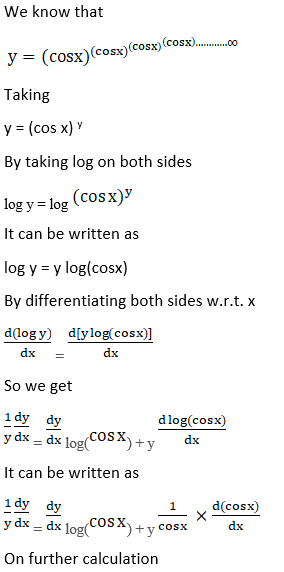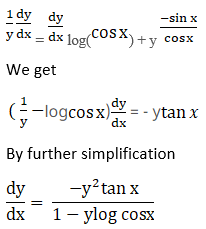3. If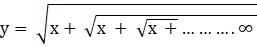, prove that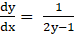.

Solution: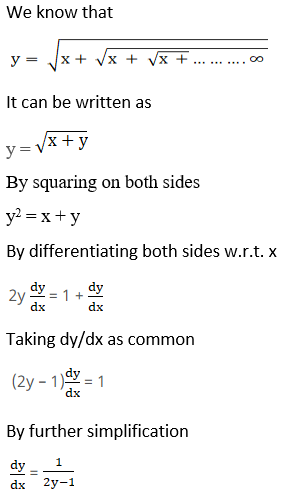4. If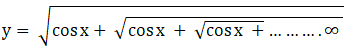, prove that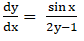.

Solution: